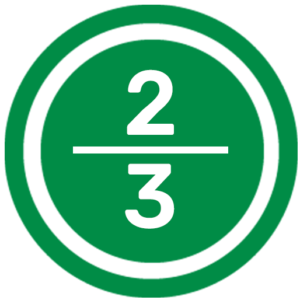# Fractions Calculator

Whether you need to add, subtract, multiply or divide fractions, the Fractions Calculator can work out all equations with fractions.

### Fractions Calculator

Reduce
Put this calculator on your website
• YesNo

The Fractions Calculator can add, subtract, multiply and divide fractions quickly and easily. Work out Equations with Fractions with the help of the free Fractions Calculator.

## Equations with the Fractions CalculatorWork out Basic Mathematical Equations with the Fractions Calculator. Simply fill in the fields of the Fractions Calculator with the relevant numbers and choose whether you want to add, subtract, multiply or divide the fractions by clicking on the appropraite symbol. After clicking the calculate button, the answer to your equation will be displayed immediately.

## Calculating irreducible fractions

The Fractions Calculator can also calculate equations with smaller or irreducible fractions (a fraction in its lowest terms or reduced fraction). To work out an equation with simplified fractions, simply click on the reduce button at the bottom of the Fractions Calculator and return to your fractions calculation. By clicking on the calculate button, the Fractions Calculator will display the answer to your equation.